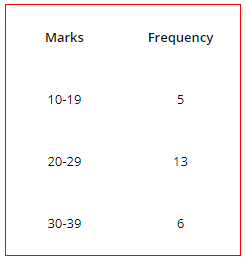# Frequency Distribution: Frequency Series, Types of Series with Examples

The compilation of these Organisation of Data Notes makes students exam preparation simpler and organised.

## Frequency Distribution

Assume you are collecting the data about the weights of all students in your class. Definitely, there will be many students that share the same weight. In such a case, we can say that some weight values occur frequently. Thus we can construct a statistical frequency series out of this data according to a frequency distribution.

### Frequency Series

A frequency distribution is the part of a broader type of statistical series, which is frequency series. A frequency series is simply a series that contains frequency. Before discussing a frequency series, there are certain terms of absolute importance which are as follows:

Frequency
Frequency is basically the number of times a data item occurs in the series. In other words, it deals with how frequent a data item is in the series. For example, if the weight of 5 students in a class is exactly 65 kg, then the frequency of data item 65kg is 5.

Class Frequency
Generally, we construct various classes that have a range of values from the data. The class frequency is the number of times the items corresponding to a class interval repeat in the series. In simple words, it is the frequency of a class. For example, if there are 10 students weighing 50-60 kg, then the class frequency for the class 50-60 is 10.

Tally Bars
We generally use tally bars to count the frequency in a series. Whenever an item occurs in a series, it is represented by a ‘|’. This is an item that occurs 4 times then it is represented as ||||. One important point to remember is that we represent the fifth occurrence by crossing the four tally bars. This is the four and cross method.Discrete Series or Frequency Array: In this type, there are no class intervals with a specific range of data items. Instead, there are data items with their exact value and corresponding frequency. Definitely, we use this when the data collected is very small.Frequency Distribution: Here, we mention various class intervals with a range of values for data intervals with their respective class frequencies. We will be studying further about below.

### Frequency Distribution

In a frequency distribution series, we make use of various class intervals to represent the range of values of the data under consideration. The class intervals are framed according to the lowest and maximum values of the given data. Also, these class intervals have an upper and lower values.

Whenever an item occupies the range between upper and lower values of a class interval, it is written against the corresponding class interval using a tally bar.

Further, a major difference between frequency array and frequency distribution series is that in frequency array the X-variable or the basis of classification (weight of students in our example) generally assumes discrete values. Whereas, in a frequency distribution, the X-variable or the basis of classification assumes continuous values.### Types of Frequency Distribution

The frequency distribution is further classified into five. These are:

Exclusive Series
In such a series, for a particular class interval, all the data items having values ranging from its lower limit to just below the upper limit are counted in the class interval. In other words, we do not include the items that have values less than the lower limit, equal to the upper limit, and greater than the upper limit.

Note that here the upper limit of a class repeats itself in the lower limit of the next interval. This is the most used type of frequency distribution.Inclusive Series
On the contrary to exclusive series, an inclusive series includes both its upper and lower limit. Of course, this means that we do not include the items with values less than the lower limit and greater than the upper limit.Open End Series
In an open-end series, the lower limit of the first class in the series and the upper limit of the last class in the series is missing. Instead, there is ‘below the lower limit’ of the first class and ‘lower limit and above the lower limit’ of the last class.Cumulative Frequency Series
In a cumulative frequency series, we either add or subtract the frequencies of all the preceding class intervals to determine the frequency for a particular class. Further, the classes are converted into either ‘less than the upper limit’ or ‘more than the lower limit’.

Mid-Values Frequency Series
A mid-value frequency series is one in which we have the mid values of class intervals and the corresponding frequencies. In other words, the mid values represent the range of a particular class interval. To determine the upper and lower limits of a class represented by its mid-value we can use the following formulas:

Lower Limit = m – (1\2) × i
Upper Limit = m – (1\2) × i

Here, m = The mid-value of the class
i = Difference between the mid-values

Example:

Question:
List the different types of frequency distribution series.